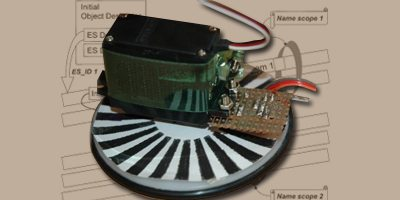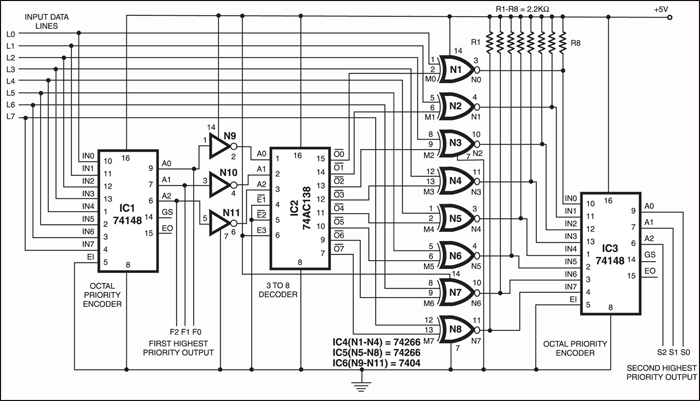# A Hierarchial Priority Encoder

This is a circuit for a priority encoder that encodes the highest and the second highest priority information of an 8 line incoming data. -- DR. BHASKARA RAON

2531Anormal priority encoder encodes only the highest-order data line. But in many situations, not only the highest but the second-highest priority information is also needed. The circuit presented here encodes both the highest-priority information as well as the second-highest priority information of an 8-line incoming data. The circuit uses the standard octal priority encoder 74148 that is an 8-line-to-3-line (4-2-1) binary encoder with active-‘low’ data inputs and outputs.The first encoder (IC1) generates the highest-priority value, say, F. The active- ‘low’ output (A0, A1, A2) of IC1 is inverted by gates N9 through N11 and fed to a 3-line-to-8-line decoder (74138) that requires active-‘high’ inputs. The decoded outputs are active-‘low’. The decoder identifies the highest-priority data line and that data value is cancelled using XNOR gates (N1 through N8) to retain the second- highest priority value that is generated by the second encoder.

To understand the logic, let the incoming data lines be denoted as L0 to L7. Lp is the highest-priority line (active-‘low’) and Lq the second-highest priority line active-‘low’). Thus Lp=0 and Lq=0. All lines above Lp and also between Lp and Lq (denoted as Lj) are at logic 1. All lines below Lq logic state are irrelevant, i.e. ‘don’t care’. Here p is the highest-priority value and q the second-highest-priority value. (Obviously, q has to be lower than p, and the minimum possible value for p is taken as ‘1’.)

Priority encoder IC1 generates binary output F2, F1, F0, which represents the value of p in active-‘low’ format. The complemented F2, F1, and F0 are applied to 3-line-to-8-line (one out of eight outputs is active-‘low’) decoder 74138. Let the output lines of 74138 be denoted as M0 through M7. Now only one line is active-‘low’ among M0 through M7, and that is Mp (where the value of p is explained as above). Therefore the logic level of line Mp is ‘0’ and that of all other M lines ‘1’.

The highest-priority line is cancelled using eight XNOR gates as shown in the figure. Let the output lines from XNOR gates be N0 through N7. Consider inputs Lp and Mp of the corresponding XNOR gate. Since Mp = 0 and also Lp = 0, the output of this XNOR gate is Np = complement of Lp = 1. All other L’s are not changed because the corresponding M’s are all 1’s. Thus data lines N0 through N7 are same as L0 through L7, except that the highest-priority level in L0 through L7 is cancelled in N0 through N7.

The highest-priority level in N0 through N7 is the second-highest priority leftover from L0 through L7, i.e. Nq=0 and Nj=1 for q

For example, let L0 through L7 = X X X 0 1 1 0 1. Here the highest ‘0’ line is L6 and the next highest is L3 (X denotes ‘don’t care’). Thus p=6 and q=3. Now the active-‘low’ output of the first priority encoder will be F2 F1 F0 = 0 0 1. The input to 74138 is 1 1 0 and it outputs M0 through M7 = 1 1 1 1 1 1 0 1. Since M6=0, only L6 is complemented by XNOR gates. Thus the outputs of XNORs are N0 through N7 = X X X 0 1 1 1 1. Now N3=0 and the highest priority for ‘N’ is 3. This value is recovered by priority encoder 2 (IC3) as S2 S1 S0 = 1 0 0.